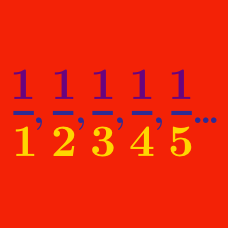Calculus

# Limits of Sequences: Level 4 Challenges

$\large \displaystyle \lim_{n \to \infty} \frac { \tan \left (89. \underbrace{9999999 \ldots 9^\circ}_{n \text{ 9's}} \right )} {\tan \left (89. \underbrace{9999999 \ldots 9^\circ}_{(n-1) \text{ 9's}} \right )} = \ ?$

A sequence adheres to the recursion relation $a_{n}=\sqrt{a_{n-1}a_{n-2}a_{n-3}}$ with initial terms $a_{0}=1,a_{1}=2,a_{2}=1$.

As $n$ tends to infinity, what is the limit of $a_n$ correct to 2 decimal places?

Evaluate

$\sqrt{\dfrac{1}{2}}\times\sqrt{\dfrac{1}{2} + \dfrac{1}{2}\sqrt{\dfrac{1}{2}}}\times\sqrt{\dfrac{1}{2} + \dfrac{1}{2}\sqrt{\dfrac{1}{2} + \dfrac{1}{2}\sqrt{\dfrac{1}{2}}}}\cdots$

The sequence $\{ {a}_{n}\}$ satisfies $\left(\frac{2n+1}{2n-1}\right)^{a_n} =e^2.$ Find $\lim_{n \rightarrow \infty}\frac{2a_n}{n+1}$.

The sequence $1, 2, 3, 2, \ldots$ has the property that every fourth term is the average of the previous three terms. That is, the sequence $\{a_n\}$ is defined as $a_1 = 1, a_2 = 2, a_3 = 3$, and $a_{n+3} = \dfrac{a_{n+2} + a_{n+1} + a_n}{3}$ for any positive integer $n$.

This sequence converges to a rational number $\frac{a}{b}$, where $a$ and $b$ are coprime positive integers. Find $a+b$.

×